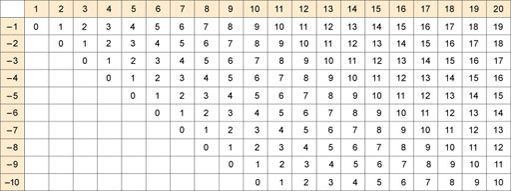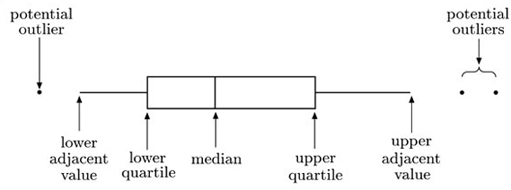## Activity 7

Tables often give information in percentages. The table below indicates how the size of households in Great Britain changed over a period of nearly 30 years.

Number of people in household1961 (%)1971 (%)1981 (%)1991 (%)
1Author(s): The Open University

## Activity 6

The table below indicates the cooling rate of tea in a teapot.

Time/mins
Author(s): The Open University

Experiments or surveys usually generate a lot of information from which it is possible to draw conclusions. Such information is called data. Data are often presented in newspapers or books.

One convenient way to present data is in a table. For instance, the nutrition panel on the back of a food packet:

### Nutrition Information

Author(s): The Open University

## Activity 1

Grateful acknowledgement is made to the following sources for permission to reproduce material in this course:

The content is taken from an activity written by Marion Hall for students taking courses in Health and Social Care, in particular those studying K101 An Introduction to Health and Social Care. The original activity is one of a set of skills activities made available to all HSC students via the HSC Resource Bank.

Except for third party materials and otherwise stated (see
Author(s): The Open University## Study another free course

There are more than 800 courses on OpenLearn for you t
Author(s): The Open University

This free course provided an introduction to studying Maths. It took you through a series of exercises designed to develop your approach to study and learning at a distance and helped to improve your confidence as an independent learner.

Author(s): The Open University

If you want to subtract without using a calculator, you need to know off by heart what you get if you subtract any number up to 10 from any bigger number up to 20. All the possible combinations are shown in the table below.Author(s): The Open University

Now that you’ve learned how to do subtraction on paper, you might want to practice your new skills.

To practice subtracting whole numbers, including borrowing where necessary, go to the Practice Subtracting section of the Numbers website and click on Get sum. Then follow the instructions.

To practise subtracting decimals, go to
Author(s): The Open University

Subtracting whole numbers such as 52 from 375 is fairly straightforward. Subtracting decimal numbers such as 6.892 from 223.6 uses the same process but with one extra step – you have to line the decimal points up first.

Rather than arranging your two numbers so that they line up on the right-hand side, you need to line up the decimal points, regardless of how many numbers there are after the decimal point. In the example below, the top number has one number after the decimal point. It
Author(s): The Open University

If the digit at the top of any column in your subtraction is smaller than the digit at the bottom of the same column, you need to borrow (or carry) from the next column on the left.

There is an example of this below. Click on each step in turn to see how to carry out the calculation.

Active content not displayed. This content req
Author(s): The Open University

If the numbers you want to subtract are too large for you to do the calculation in your head, you can use a calculator. Alternatively, you can do the calculation on paper.

Write your starting number at the top with the number you want to subtract from it underneath. Because the order in which you subtract one number from another matters, it is important to put the correct numbers on top and bottom. Then draw a line underneath.

Write the numbers so the digits form columns and they
Author(s): The Open University

After studying this course, you should be able to:

• subtract one number from another

• subtract using decimals

• practise your subtraction skills.

Author(s): The Open University

Instead of putting the two left over biscuits back in the tin, you might instead decide to break them into halves and then give one of the resulting four halves to each person so that everyone receives a total of one and a half biscuits. In this case, the answer has to be expressed as a fraction or the equivalent decimal number:

• 6 ÷ 4 = 1½
• 6 ÷ 4 = 1.5

A fraction is really just another way of expressing a division because ½ means
Author(s): The Open University

An important thing to remember about division is that it has different rules from multiplication.

For example when you multiply two numbers together, it doesn’t matter what order you multiply them in. So 8 x 4 is exactly the same as 4 x 8. The result is 32 in both cases.

But in division, order matters. So 8 ÷ 4 is different from 4 ÷ 8.

The answer to the first is 2. If you multiply 4 by 2 you get 8.

But the answer to the second is 0.5 (a half). If you multiply 8 by
Author(s): The Open University

There are practically no new theories or new principles in this section. We shall work through some examples, and you will see how basic techniques and approaches that you have already learned can be combined to allow you to use tabular data efficiently.

## Example 3.1 Health personnel in Thailand

Author(s): The Open University

In this section you have been introduced to the boxplot. This is a graphic that represents the key features of a set of data. A typical boxplot is shown in Figure 1.8.Author(s): The Open University

It is worth noting that a special term is reserved for the square of the sample standard deviation: it is known as the sample variance.

## The sample variance

The sample variance of a data sample x 1, x 2, …, xn
Author(s): The Open University5.6 Quartiles and the interquartile range

The first alternative measure of dispersion we shall discuss is the interquartile range: this is the difference between summary measures known as the lower and upper quartiles. The quartiles are simple in concept: if the median is regarded as the middle data point, so that it splits the data in half, the quartiles similarly split the data into quarters. This is, of course, an over-simplification. With an even number of data points, the median is defined to be the average of the middle two: de
Author(s): The Open University